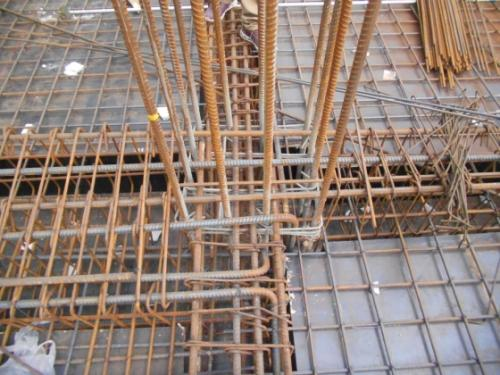# 常用钢筋理论重量表，建议收藏0.617是圆10钢筋每米重量。钢筋重量与直径（半径）的平方成正比。

G/m=0.617*D*D/100

Φ12(含12)以下和Φ28(含28)的钢筋一般小数点后取三位数，Φ14至Φ25钢筋一般小数点后取二位数

Φ6=0.222Kg

Φ8=0.395Kg

Φ10=0.617Kg

Φ12=0.888Kg

Φ14=1.21Kg

Φ16=1.58Kg

Φ18=2Kg

Φ20=2.47Kg

Φ22=3Kg

Φ25=3.86Kg

Φ28=4.83Kg

Φ32=6.31Kg

Φ36=7.99Kg

Φ40=9.87Kg

1、角钢、工字钢和槽钢的准确计算公式很繁，表列简式用于计算近似值。

2、f值：一般型号及带a的为3.34，带b的为2.65，带c的为2.26。

3、e值：一般型号及带a的为3.26，带b的为2.44，带c的为2.24。

4、各长度单位均为毫米。

##### 装修达人
•毛爱斌
• 翻新

• 老房

• 空间

• 资深

翻新达人 毛爱斌 向TA咨询
•胡治平
• 大咖

• 收纳

• 日系

设计达人 胡治平 向TA咨询
•李慧慧
• 软装

• 收纳

• 别墅

软装达人 李慧慧 向TA咨询
•李坤
• 大咖

• 空间

• 沟通

改造达人 李坤 向TA咨询

1分钟 获取报价